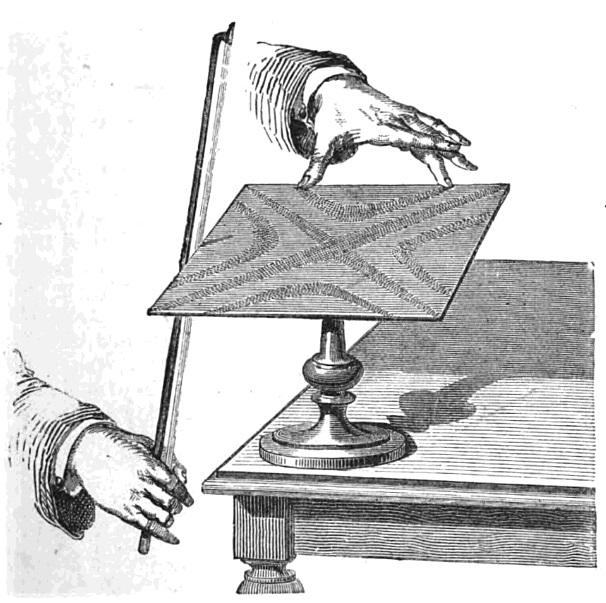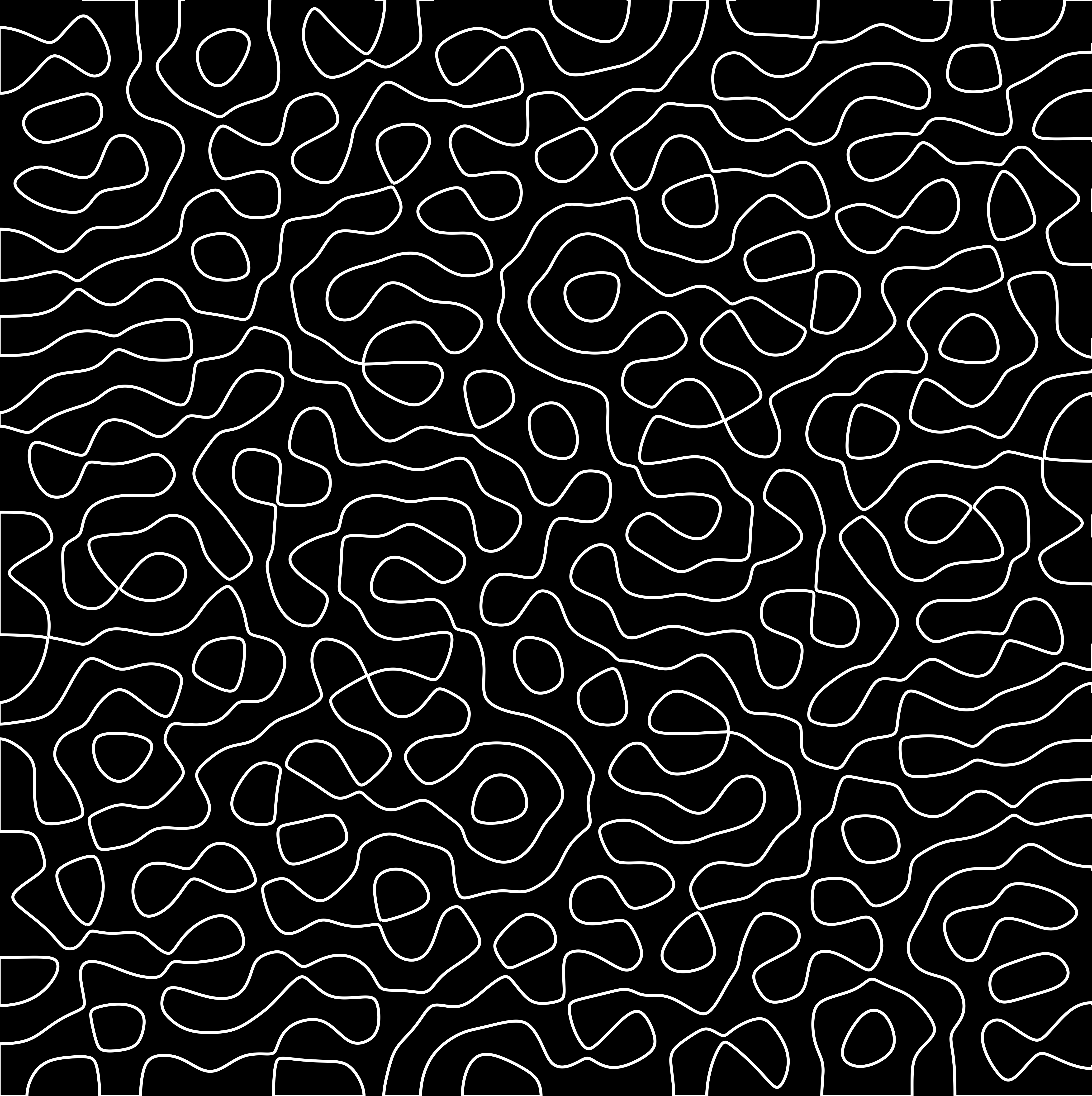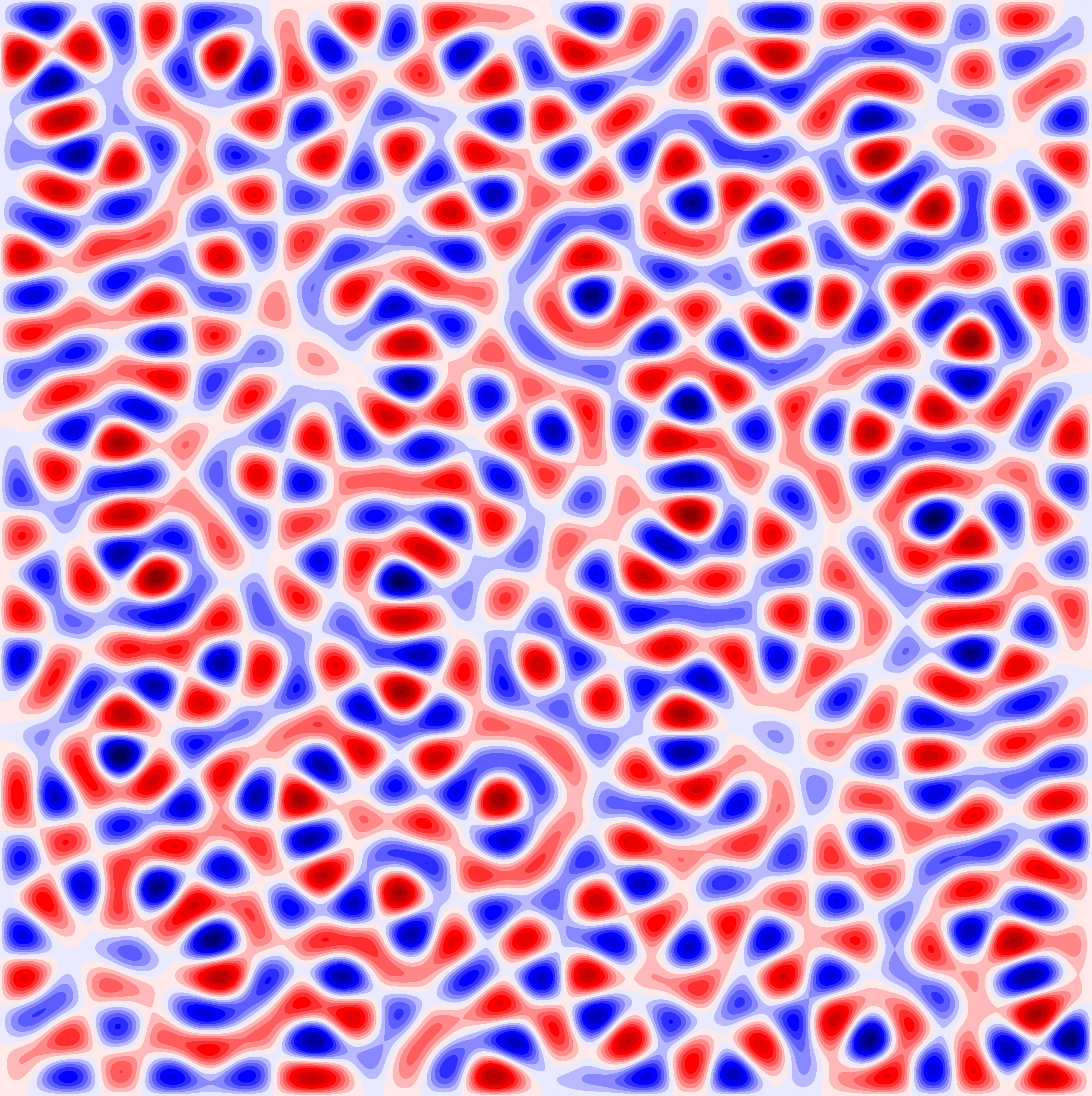# On the cover: Chladni figures of a square drum

Find out more about the patterns on the cover of Issue 07The 18th century—the age of enlightenment. Ernst Chladni travelled around Europe demonstrating his ‘musical curiosities’. The star attraction was a novel technique to expose the various modes of vibration of a rigid surface.Chladni’s original method to expose vibrational modes of a rigid plate

A plate or membrane, when resonating, divides into regions that vibrate in opposite directions. These are bounded by fixed lines where no vibration occurs (nodal lines). Chladni would lightly scatter sand on a metal plate, then violin-bow the plate to the point of resonance. The vibration encouraged the sand to accumulate upon the nodal lines, and patterns—now called Chladni figures—emerged.

Forget rigid plates (they’re so 18th century). Let’s clamp the edges of a flexible square membrane to make a square drum. Suppose you scatter fine sand (or… chalkdust) on it and then vibrate at prescribed frequencies. Equally fascinating nodal patterns emerge—the Chladni figures of a square drum.

So far this all seems dangerously close to real life. This is a maths magazine. Where’s the maths?The modes of vibration for the square can be uniquely described by the surfaces that they draw out at their maximal amplitude. By analogy think of a vibrating (one dimensional) string. When vibrating, the whole string oscillates from a sinusoidal curve, to the negative of the curve, and back again. The situation is very similar in two dimensions, except we have a surface on a square domain, instead of a curve on an interval. The modes of vibration are stationary solutions of a differential equation called the wave equation. When you solve this equation, it turns out that there are only special, isolated, frequencies at which standing waves can form on the drum. We call the set of these special values the frequency spectrum of the square drum.

Remarkably, for each fixed frequency in this spectrum there are multiple basic vibrational modes. By superimposing amplified versions of these basic modes, we can form any and every vibrational mode of that frequency. Even more remarkably, the multiplicity is intimately related to a tricky arithmetic function which is notorious in number theory.Chladni figure with nodal lines traced out in white

Now, back to nodal lines. The Chladni figure of each basic vibrational mode for the square is, by itself, easy to understand. It looks similar to the simplest of the experimental patterns we saw above—a grid of lines. However, a superposition of equal-frequency vibrational modes can produce strikingly intricate and complex nodal patterns. Even the most basic geometric question—How many distinct nodal lines are there in a ‘typical’ pattern?—is considerably difficult to answer with mathematical rigour.

The first step towards answering this question is to put ‘typical’ on firm mathematical footing. And that brings us to (possibly) the only question you really care about right now: So what exactly are those patterns, tiling the cover of Issue 07?Contour plot coloured $\text{red}>0$, $\text{white}=0$, $\text{blue}<0$

Pick a frequency $\lambda$ from the spectrum of the square drum, list all of the basic vibrational modes of frequency $\lambda$, amplify each of them by a random (independent and standard normally-distributed) coefficient, sum them together, and what comes out looks something like the contour plot below right. The superposition of randomly-amplified basic vibrational modes is usually called a (boundary-adapted) arithmetic random wave, and the plot is a sample from this random wave. My research focuses on studying the statistical properties of the number of distinct nodal lines—a random variable which is defined by the random wave.

We could think of this coloured square as a (randomly generated) sinusoidal landscape with a preference for oscillating around sea-level. From this topographical perspective, the nodal lines are the contour lines at sea-level of the landscape. The image to the right is a sample from the boundary-adapted arithmetic random wave with $\lambda =1105\mathrm{\pi}^2$.

Why study such an obscure mathematical object?

Well: they have interesting and subtle statistical properties, not to mention that the  sample waves look pretty damn fine. But if that isn’t enough for you, the nodal patterns of boundary-adapted arithmetic random waves are of interest to a much wider group than mathematicians. Quantum physicists like them too: they capture properties of the wavefunctions of a ‘quantum billiard ball trapped in a box’ with a fixed energy corresponding to the frequency of the wave. And the community of interest is  even wider than the combined esoteric worlds of quantum and mathematics. Earthquake scientists; makers of fine musical instruments; all are interested in the properties of nodal lines—the sets which remain stationary when a domain is vibrating at resonant frequencies.

More than just pretty patterns, I hope you agree.James Cann is a PhD student at UCL and is attached to the London School of Geometry & Number Theory. He likes maths best when explained with pictures.
+ More articles by James